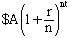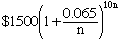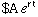Name: Gina Who is asking: Teacher Level: Secondary Question: I'm tutoring an Algebra II student and we're having trouble with this one: You deposit \$1500 in an account that pays 6.5% annual interest, compounded continuously. Find the balance after 10 years. I'm not sure what to do with the "compounded continuously" part. Hi Gina, If you invest \$A at an interest rate of r for t years, compounded n times per year then the balance after the t years isWith the example that you give the balance after 10 years would beIt's an interesting calculator exercise to see what happens as you increase n. For simple interest n = 1, or you might compount twice per year (n = 2) or every day (n = 365) or every minute (n = 365x24x60) or ... What you will find is that as n increases the balance approaches \$2873.3099872... This is the balance that results from "compounding continuously". The expression that returns this balance immediately iswhere e is the base for the natural logarithms (e is approximately 2.71828) I hope this helps, Penny Go to Math Central